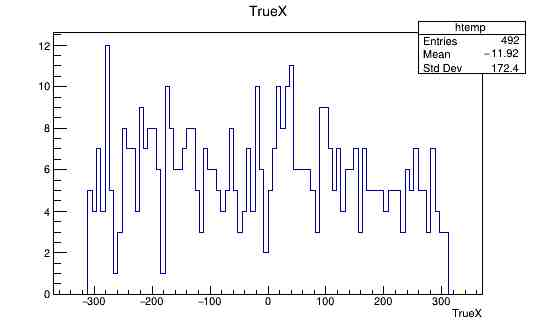# Adding a branch to a tree and saving

Hello; I’m trying to add a branch to a tree from a file.root and then I want to save the file, i wrote the following:

``````void TreeAdd(){
TFile *fp = new TFile(FileName.c_str(), "update");
if (fp->IsZombie()) {
std::cout << "Error opening file" << std::endl;
exit(-1);
}
TTree *T = (TTree*) fp -> Get(TreePathInFolder.c_str());
Float_t px;
Float_t pTest;
std::string temp = TestBranchName + std::string("/F");
TBranch *pTestBranch = T->Branch(TestBranchName.c_str(), &pTest, temp.c_str());
for (Int_t i = 0; i<(nentries); i=i+2) {
T -> GetEntry(i);
pTest = px;
pTestBranch -> Fill();
}
T -> Write("",TObject::kOverwrite);
T -> Print();
fp -> Save();
fp -> Close();
}
``````

Now T->Print() does show the modified tree with the added branch but when I reopen the file the tree is not modified, why is this happening?

At least:
`for (Long64_t i = 0; i < T->GetEntries(); i++) {`

nentries is T->GetEntries() and I need the for loop to go over only half of the entries. The additional branch gets created so that part of the code should work; the issue is with saving the file

I found out that the problem is that instead of adding the new branches to the tree it creates a new tree in the root path of the file that I opened with fp; so the question is: how do I tell my program to add the brances to the existing tree instead of creating a new one?

Since you don’t show all the relevant/related code, it’s hard to tell what exactly you are doing. Anyway, one thing to try is to add

``````  fp->cd();
``````

just before doing `T->Write();`. Also, I might be wrong but I don’t think `fp->Save()` exists; in any case it is not needed.

Try:

``````fp->cd("your/folder/with/tree");
TTree *T; gDirectory->GetObject("tree_name", T);
``````

Found the solution for the tree situation:

``````  fp -> cd(FolderNames[SelectedFolder].c_str());
TTree *T;
gDirectory->GetObject(TreeNames.c_str(), T);
...
T -> Write("", TObject::kOverwrite);
``````

But now I have a new problem, this is the full code:

``````
#define NTrees 4
#define NFolders 4
#define NPlots 2
#define XMin -400.0
#define XMax 400.0
#define YMin -5.0
#define YMax 30000.0

//Change this to change the used folder
#define SelectedFolder 0
#define SelectedTree 0

//Theese are the folder inside the file
std::string FolderNames[NFolders] = {"flashmatchAnaArOnly", "flashmatchAnaXeOnly", "flashmatchAna10ppm"};

//Change this to change the selected leaf
std::string OriginalLeafName = "TrueX";

std::string TestLeafName = OriginalLeafName + std::string("_test");
std::string FitLeafName = OriginalLeafName + std::string("_fit");

//Change this to change the file from which the tree is loaded
std::string FileName = "../Output/OutAna_500Events_100MeV.root";

//Trees inside each folder
std::string TreeNames[NTrees] = {"FlashMatchTree" ,"LargestFlashTree", "SelectedFlashTree", "CountWaveforms"};

void TreeUpdate(){
TFile *fp = new TFile(FileName.c_str(), "UPDATE");
//Check if file is opened
if (fp->IsZombie()) {
std::cout << "Error opening file" << std::endl;
exit(-1);
}
std::string TreePathInFolder = std::string("/") + FolderNames[SelectedFolder]+std::string("/")+TreeNames[SelectedTree];
fp -> cd(FolderNames[SelectedFolder].c_str());
TTree *T;
gDirectory->GetObject(TreeNames.c_str(), T);

Float_t px = 0;
Float_t pTest = 0;
Float_t pFit = 0;
//
TBranch *OriginalBranch = T -> GetBranch(OriginalLeafName.c_str());
OriginalBranch->SetAutoDelete(kTRUE);
//
pTest=0;
pFit=0;
TBranch *pTestBranch = T->Branch(TestLeafName.c_str(), &pTest, "pcs/F");
TBranch *pFitBranch = T->Branch(FitLeafName.c_str(), &pFit, "pys/F");
//
Long64_t nentries = T->GetEntries();
//
Float_t v1[nentries];
//
pTest=0;
pFit=0;
for (Int_t i = 0; i<nentries; i++) {
T -> GetEntry(i);
if(i%2 == 0){
pTest = px;
pTestBranch -> Fill();
}
else{
pFit = px;
pFitBranch -> Fill();
}
}
//
pTest=0;
pFit=0;

T -> Write("", TObject::kOverwrite);
T -> Print();
T->Draw(TestLeafName.c_str());
fp -> Close();
}
``````

I’m trying to take the even and odds entries of my leaf and copy them in two different branches TestLeaf and FitLeaf; this code doesn’t give any error in compilation, the `T->Print()` shows the added branches with the right number of entries but `Draw(TestLeafName.c_str());`gives an histogram with an additional column as high as the number of entries; I don’t understand what I’m doing wrong at allAll tree branches (i.e., “old” and “new”) must have the same numbers of entries.

So my best bet is to make a new tree with only the new branches, right?

``````  // ...
for (Int_t i = 0; i<nentries; i++) {
T -> GetEntry(i);
if(i%2 == 0){
pTest = px;
pTestBranch -> Fill();
pFit = -9999.;
pFitBranch -> Fill();
}
else{
pTest = -9999.;
pTestBranch -> Fill();
pFit = px;
pFitBranch -> Fill();
}
}
// ...
T->Draw(TestLeafName.c_str(), (TestLeafName + " > -9999.").c_str());
// ...
``````

Thank you very much!